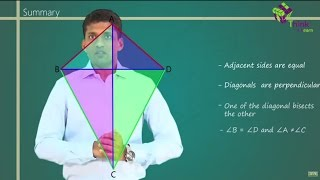Quadrilateral is a 4 sided polygon bounded by 4 finite line segments. A quadrilateral has 2 diagonals based on which it can be classified into concave or convex quadrilateral. In case of convex quadrilaterals, diagonals always lie inside the boundary of the polygon.

Based on the lengths of sides and angles, common convex quadrilaterals are:

1. Trapezoid
2. Parallelogram
3. Square
4. Rectangle
5. Rhombus
6. Kite

Let us discuss in brief the properties of quadrilaterals.

1. Properties of Parallelogram :
2. Opposite sides are parallel and equal to each other
3. Opposite angles are equal
4. Diagonals bisect each other
5. Diagonals divide the parallelogram into two congruent triangles
6. If any one of the angles is $90^{\circ}$, then all angles are right angles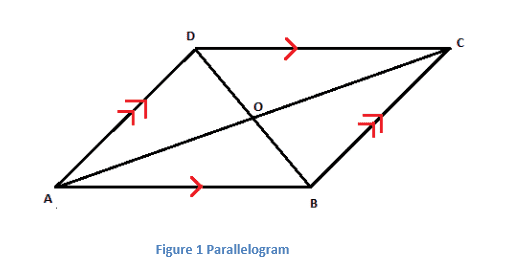1. Properties of Kite:
• Two distinct pairs of adjacent sides are congruent
• Diagonals of a kite intersect at right angles
• One of the diagonals is the perpendicular bisector of another
• Angles between unequal sides are equal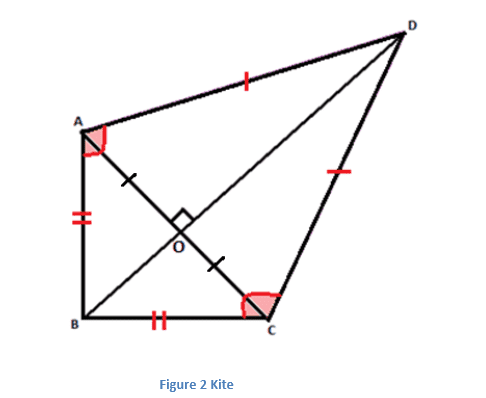1. Properties of Rhombus:
• All sides are congruent
• Diagonals are perpendicular bisectors of each other
• Each diagonal isthe angle bisector of both the opposite angles
• Every rhombus is a parallelogram and a kitewith all sides of equal length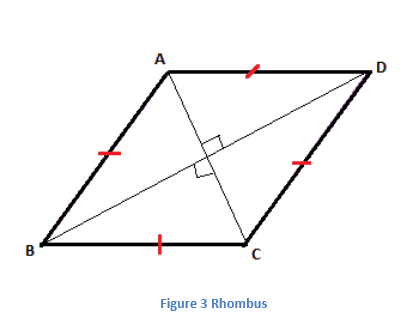1. Properties of Rectangle:
• All angles in a rectangle are right angles
• Opposite sides are equal in a rectangle
• Diagonals are congruent and bisect each other
• Every rectangle is a parallelogram with at least one right angle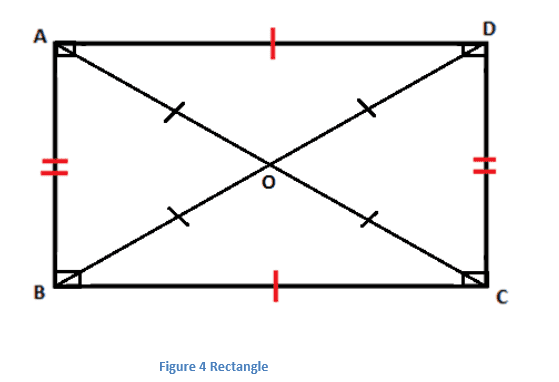1. Properties of Square:
• All sides are congruent
• Each interior angle measures $90^{\circ}$<
• Diagonals are equal and are perpendicular bisectors of each other
• Every square is a parallelogram in which diagonals are congruent and bisect the angles
• Every square is a rectangle and a rhombus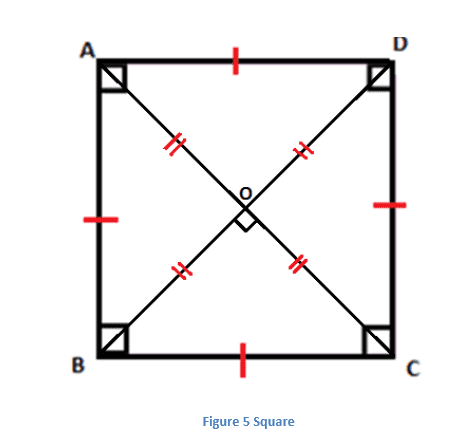1. Properties of Trapezoid:
• One pair of opposite sides is parallel
• Diagonals intersect each other in the same ratio
• Two adjacent angles are supplementary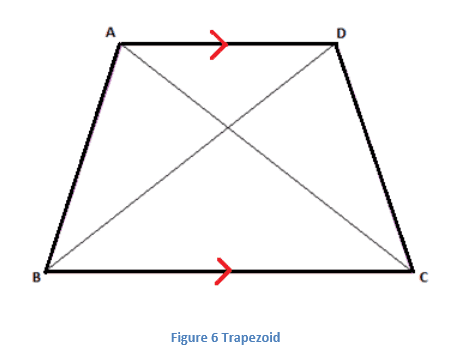#### 1 Comment

1. Anita Handa

I am a private teacher. I was looking for properties of all quadrilateral, so that I could give them to my kids. Like notes and your site was very helpful.